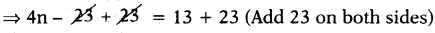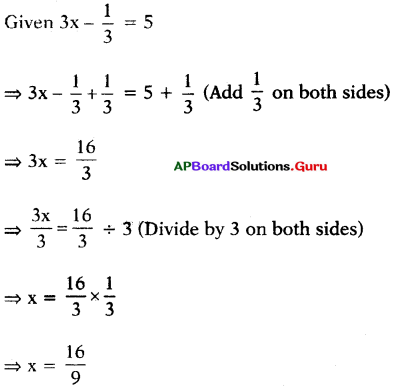# HSSlive: Plus One & Plus Two Notes & Solutions for Kerala State Board

## AP Board Class 7 Maths Chapter 3 Simple Equations Ex 3.2 Textbook Solutions PDF: Download Andhra Pradesh Board STD 7th Maths Chapter 3 Simple Equations Ex 3.2 Book AnswersAP Board Class 7 Maths Chapter 3 Simple Equations Ex 3.2 Textbook Solutions PDF: Download Andhra Pradesh Board STD 7th Maths Chapter 3 Simple Equations Ex 3.2 Book Answers

## Andhra Pradesh State Board Class 7th Maths Chapter 3 Simple Equations Ex 3.2 Books Solutions

 Board AP Board Materials Textbook Solutions/Guide Format DOC/PDF Class 7th Subject Maths Chapters Maths Chapter 3 Simple Equations Ex 3.2 Provider Hsslive

2. Click on the Andhra Pradesh Board Class 7th Maths Chapter 3 Simple Equations Ex 3.2 Answers.
3. Look for your Andhra Pradesh Board STD 7th Maths Chapter 3 Simple Equations Ex 3.2 Textbooks PDF.
4. Now download or read the Andhra Pradesh Board Class 7th Maths Chapter 3 Simple Equations Ex 3.2 Textbook Solutions for PDF Free.

## AP Board Class 7th Maths Chapter 3 Simple Equations Ex 3.2 Textbooks Solutions with Answer PDF Download

Find below the list of all AP Board Class 7th Maths Chapter 3 Simple Equations Ex 3.2 Textbook Solutions for PDF’s for you to download and prepare for the upcoming exams:

Question 1.
Give the steps you will use to separate the variables and then solve the equation.
(i) 5𝑚3 = 10
Given 5𝑚3 = 10
⇒ 5𝑚3 × 3 = 10 × 3 (Multiply with 3 on both sides)
⇒ 5m = 30
⇒ 5𝑚5 = 305 (Divide both sides by 5)
⇒ m = 6

Check:
Substitute m = 6 in the given equation,
LHS = 5𝑚3 = 5(6)3 = 303 = 10 = RHS.
Hence verified.

(ii) 4M – 23 = 13
Given 4n – 23 = 13⇒ 4n = 36
⇒ 4𝑛4 = 364 (Divide both sides by 4)
⇒ n = 9

Check:
Substitute n = 9 in the given equation.
LHS = 4n – 23
= 4(9) – 23
= 36 – 23 = 13 = RHS
Hence verified.

(iii) – 5 + 3x = 16
Given – 5 + 3x = 16
⇒ – 5 + 3x + 5 = 16 + 5 (Add 5 on both sides)
⇒ 3x = 21
⇒ 3𝑥3 = 213 (Divide by 3 on both sides)
⇒ x = 7

Check:
Substitute x = 7 in the given equation
LHS = – 5 + 3x
= – 5 + 3(7)
= – 5 + 21 = 16 = RHS
Hence verified.

(iv) 2(y – 1) =8
Given 2(y – 1) = 8
⇒ 2y – 2 = 8 (Distributive property)
⇒ 2y – 2 + 2 = 8 + 2 (Add 2 on both sides)
⇒ 2y = 10
⇒ 2𝑦2 = 102 (Divide by 2 on both sides)
⇒ y = 5

Check: Substitute y = 5 in the given equation
LHS = 2(y – 1)
= 2(5 – 1)
= 2 × 4 = 8 = RHS
Hence verified.

Question 2.
Solve the following simple equations and check the results.
(i) 3x = 18
Given 3x = 18
⇒ 3𝑥3 = 183 (Divide by 3 on both sides)
⇒ x = 6

Check: Substitute x = 6 in 3x = 18
LHS ⇒ 3x = 3(6) = 18 = RHS
Hence verified.

(ii) 𝑏7 = – 2
Sol.
Given 𝑏7 = – 2
⇒ 𝑏7 × 7 = – 2 × 7 (Multiply by 7 on both sides)
⇒ b = – 14

Check: Substitute b = – 14 in
𝑏7 = – 2
LHS = 𝑏7 = 147 = – 2 = RHS
Hence verified

(iii) – 2x = – 10
Given – 2x = – 10
⇒ 2𝑥2 = 102 (Divide by – 2 on both sides)
⇒ x = 5

Check:
Substitute x = 5 in
– 2x = – 10
LHS = – 2x
= – 2(5) = – 10 = RHS
Hence verified.

(iv) 10 + 6a = 40
Given 10 + 6a = 40
⇒ 10 + 6a – 10 = 40 – 10 (Subtract 10 on both sides)
⇒ 6a = 30
⇒ 6𝑎6 = 306 (Divide by 6 on both sides)
⇒ a = 5

Check: Substitute a = 5 in 10 + 6a = 40
LHS = 10 + 6a
= 10 + 6(5)
= 10 + 30
= 40 = RHS
Hence verified.

(v) – 7m = 21
Given – 7m = 21
⇒ 7𝑚7 = 217 (Divide by – 7 on both sides)
⇒ m = – 3

Check:
Substitute m = in – 7m = 21
LHS = – 7m
= – 7 (- 3)
= 21 = RHS
Hence verified.

(iv) 4p + 7 = – 21
Given 4p + 7 = – 21
⇒ 4p + 7 – 7 = – 21 – 7 (Subtract 7 on both sides)
⇒ 4p = – 28
⇒ 4𝑝4 = 284 (Divide by 4 on both sides)
⇒ p = – 7

Check:
Substitute p = – 7 in 4p + 7 = – 21
LHS = 4p + 7
= 4(- 7) + 7
= – 28 + 7
= – 21 = RHS
Hence verified.

(vii) 3x – 13 = 5Check: Substitute x = 169 in 3x – 13 = 5
LHS = 3x – 13
= 3(169) – 13
16313
1613 = 153
= 5 = RHS
Hence verified.

(viii) 18 – 7n = – 3
Given 18 – 7n = – 3
⇒ 18 – 7n – 18 = – 3 – 18 (Subtract 18 on both sides)
⇒ – 7n = – 21
⇒ 7𝑛7𝑛 = 217 (Divide by – 7 on both sides)
⇒ n = 3

Check:
Substitute n = 3 in 18 – 7n = – 3
LHS = 18 – 7n
= 18 – 7(3)
= 18 – 21
= – 3 = RHS
Hence verified.

(ix) 3(k + 4) = 21
Sol.
Given 3(k + 4) = 21
⇒ 3k + 12 = 21 (Distributive property)
⇒ 3k + 12 – 12 = 21 – 12 (Subtract 12 on both sides)
⇒ 3k = 9
⇒ 3k3 = 93 (Divide by 3 on both sides)
⇒ k = 3

Check:
Substitute k = 3 in 3(k + 4) = 21
LHS = 3(k + 4)
= 3(3 + 4)
= 3 × 7 = 21= RHS
Hence verified.

(x) 9 (a + 1) + 2 = 11
Given 9(a + 1) + 2 = 11
⇒ 9(a + 1) + 2 – 2 = 11 – 2 (Subtract 2 on both sides)
⇒ 9(a + 1) = 9
⇒ 9(𝑎+1)9 = 99 (Divide by 9 on both sides)
⇒ a + 1 = 1
⇒ a + 1 – 1 = 1 – 1 (Subtract 1 on both sides)
⇒ a = 0

Check:
Substitute a = 0 in 9(a + 1) + 2 = 11
LHS = 9(a + 1) + 2
= 9(0 + 1) + 2
= 9(1) + 2
= 9 + 2 = 11 = RHS
Hence verified

## Andhra Pradesh Board Class 7th Maths Chapter 3 Simple Equations Ex 3.2 Textbooks for Exam Preparations

Andhra Pradesh Board Class 7th Maths Chapter 3 Simple Equations Ex 3.2 Textbook Solutions can be of great help in your Andhra Pradesh Board Class 7th Maths Chapter 3 Simple Equations Ex 3.2 exam preparation. The AP Board STD 7th Maths Chapter 3 Simple Equations Ex 3.2 Textbooks study material, used with the English medium textbooks, can help you complete the entire Class 7th Maths Chapter 3 Simple Equations Ex 3.2 Books State Board syllabus with maximum efficiency.

## FAQs Regarding Andhra Pradesh Board Class 7th Maths Chapter 3 Simple Equations Ex 3.2 Textbook Solutions

#### Can we get a Andhra Pradesh State Board Book PDF for all Classes?

Yes you can get Andhra Pradesh Board Text Book PDF for all classes using the links provided in the above article.

## Important Terms

Andhra Pradesh Board Class 7th Maths Chapter 3 Simple Equations Ex 3.2, AP Board Class 7th Maths Chapter 3 Simple Equations Ex 3.2 Textbooks, Andhra Pradesh State Board Class 7th Maths Chapter 3 Simple Equations Ex 3.2, Andhra Pradesh State Board Class 7th Maths Chapter 3 Simple Equations Ex 3.2 Textbook solutions, AP Board Class 7th Maths Chapter 3 Simple Equations Ex 3.2 Textbooks Solutions, Andhra Pradesh Board STD 7th Maths Chapter 3 Simple Equations Ex 3.2, AP Board STD 7th Maths Chapter 3 Simple Equations Ex 3.2 Textbooks, Andhra Pradesh State Board STD 7th Maths Chapter 3 Simple Equations Ex 3.2, Andhra Pradesh State Board STD 7th Maths Chapter 3 Simple Equations Ex 3.2 Textbook solutions, AP Board STD 7th Maths Chapter 3 Simple Equations Ex 3.2 Textbooks Solutions,
Share: